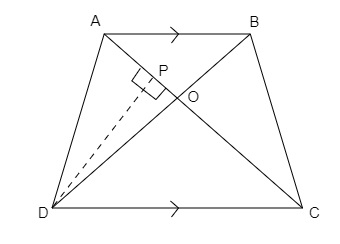# ABCD is a trapezium in which $A B \| C D$. The diagonals $A C$ and $B D$ intersect at $O .$ If $O A=6 \mathrm{~cm}, O C=8 \mathrm{~cm}$ find $\frac{\text { Area }(\Delta A O B)}{\text { Area }(\Delta C O D)}$.

Given:

ABCD is a trapezium in which $A B \| C D$. The diagonals $A C$ and $B D$ intersect at $O .$

$O A=6 \mathrm{~cm}, O C=8 \mathrm{~cm}$.

To do:

We have to find $\frac{\text { Area }(\Delta A O B)}{\text { Area }(\Delta C O D)}$.

Solution:$AB \parallel CD$

In $\triangle AOB$ and $\triangle COD$,

$\angle AOB=\angle COD$   (Vertically opposite angles)

$\angle BAO=\angle DCO$    (Alternate angles)

Therefore,

$\triangle AOB \sim\ \triangle COD$   (By AA similarity)

We know that,

If two triangles are similar, then the ratio of the area of both triangles is proportional to the square of the ratio of their corresponding sides.

This implies,

$\frac{ar(\triangle AOB)}{ar(\triangle COD)}=\frac{OA^2}{OC^2}$

$=\frac{(6)^2}{(8)^2}$

$=\frac{36}{64}$

$=\frac{9}{16}$

Therefore,

$\frac{ar(\triangle AOB)}{ar(\triangle COD)}=\frac{9}{16}$.

Updated on: 10-Oct-2022

40 Views